# GSEB Solutions Class 7 Maths Chapter 3 Data Handling InText Questions

Gujarat Board GSEB Textbook Solutions Class 7 Maths Chapter 3 Data Handling InText Questions and Answers.

## Gujarat Board Textbook Solutions Class 7 Maths Chapter 3 Data Handling InText QuestionsTry These (Page 59)

Question 1.
Weigh (in kg) at least 20 children (girls and boys) of your class. Organise the data, and answer the following questions using this data.
(i) Who is the heaviest of all?
(ii) What is the most common weight?
(iii) What is the difference between your weight and that of your best friend!
Solution:
We assume that weights (in kg) of 20 children of the class are as follows:
27, 30, 28, 24, 25, 26, 21, 20, 18, 15,
28, 16, 27, 23, 24, 26, 22, 31, 18, 26
Organising the data in ascending order, we have:
15, 16, 18, 18, 20, 21, 22, 23, 24, 24,
25, 26, 26, 26, 27, 27, 28, 28, 30, 31
(i) The child having weight 31 kg is the heaviest of all.
(ii) The most common weight is 26 kg.
(iii) If my weight is 25 kg and my friend’s weight is 27 kg, then required difference = 27 kg – 25 kg = 2 kgTry These (Page 61)

Question 1.
How would you find the average of your study hours for the whole week?
Solution:
In a week there are 7 days. The number of study hours everyday are different. Let the study hours for different days of the week are as follows:

Monday : 6 hours
Tuesday : 7 hours
Wednesday : 6 hours
Thursday : 8 hours
Friday : 8 hours
Saturday : 7 hours
Sunday : 7 hours

Total number of study hours = 6 + 7 + 6 + 8 + 8 + 7 + 7 = 49 hours
Number of total days = 7
∴ Arithmetic mean == $$\frac { 49 }{ 7 }$$ = 7
Thus, average study hours = 7 hours per day.

Try These (Page 61)

Question 1.
Find the mean of your sleeping hours during one week.
Solution:
Let the sleeping hours be as follows:

Monday : 8 hours
Tuesday : 9 hours
Wednesday : 11 hours
Thursday : 8 hours
Friday : 10 hours
Saturday : 9 hours
Sunday : 8 hours

Sum of sleeping hours
= 8 + 9+ 11 + 8 + 10+ 9 + 8 = 63
Number of days = 7
∴ Arithmetic mean =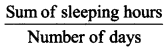= $$\frac { 63 }{ 7 }$$ hours
= 9 hours

Question 2.
Find at least 5 numbers between $$\frac { 1 }{ 2 }$$ and $$\frac { 1 }{ 3 }$$.
Solution:
The arithmetic mean of two given numbers is average between them.Thus, 5 numbers between $$\frac { 1 }{ 2 }$$ and $$\frac { 1 }{ 3 }$$ are $$\frac { 5 }{ 12 }$$, $$\frac { 11 }{ 24 }$$, $$\frac { 3 }{ 8 }$$ and $$\frac { 23 }{ 48 }$$ and $$\frac { 19 }{ 48 }$$Try These (Page 65)

Question 1.
Find the mode of
(i) 2, 6, 5, 3, 0, 3, 4, 3, 2, 4, 5, 2, 4
(ii) 2, 14, 16, 12, 14, 14, 16, 14, 10, 14, 18, 14
Solution:
(i) Given set of data is:
2, 6, 5, 3, 0, 3, 4, 3, 2, 4, 5, 2, 4
Writing the numbers with same value together, we have:
0, 2, 2, 2, 3, 3, 3, 4, 4, 4, 5, 5, 6
∵ 2, 3 and 4 occur in the given data highest times (3 times).
∴ Modes of the set of data are 2, 3 and 4.

(ii) Given set of data is:
2, 14, 16, 12, 14, 14, 16, 14, 10, 14, 18, 14
Writing the numbers with same value together, we have:
2,. 10, 12, 14, 14, 14, 14, 14, 14, 16, 16, 18
∵ 14 occurs highest times (i.e. six times).
∴ Required mode is 14.

Think, Discuss and write (Page 65)

Question 1.
Can a set of numbers have more than one model
Solution:
Yes.

Try These (Page 65 and 66)

Question 1.
Find the mode of the following data:
12, 14, 12, 16, 15, 13, 14, 18, 19,
12, 14, 15, 16, 15, 16, 16, 15, 17,
13, 16, 16, 15, 15, 13, 15, 17, 15,
14, 15, 13, 15, 14.
Solution:
Here, the given set of data has large number of observations. Therefore, we put them in a table.
∵ The highest frequency (10) is corresponding to 15.
∴ The required mode is 15.

Question 2.
Heights (in cm) of 25 children are given below:
168, 165, 163, 160, 163, 161,
162, 164, 163, 162, 164, 163,
160, 163, 160, 165, 163, 162,
163, 164, 163, 160, 165, 163,
162
What is the mode of their heights? What do we understand by mode here?
Solution:
Putting the data in a tabular form, we have: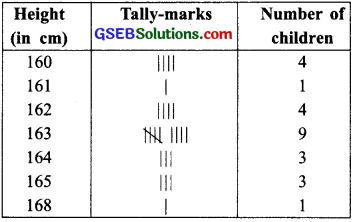Since, 163 is occurring the maximum number of times.
∴ Mode is 163 cm.
By the mode, we mean that most of students have height 163 cm.

Note : Mode is modal value of a group which occurs most frequently in the data whereas mean gives us the average of all observations of the data.Try These (Page 66)

Question 1.
Discuss with your friends and give:
(a) Two situations where mean would be an appropriate representative value to use, and
(b) Two situations where mode would be an appropriate representative value to use.
Solution:
(a) (i) For measures of heights of all students of a class, and
(ii) For weights of wheat stored in similar containers, the mean would give us a better picture of the data.

(b) (i) If we are collecting the shoe size suitable for a particular age, and
(ii) A shopkeeper selling shirts needs to know suitable size to enrich the stock, then mode is more useful.Try These (Page 67)

Question 1.
Your friend found the median and the mode of a given data. Describe and correct your friend’s error if any:
35, 32, 35, 42, 38, 32, 34
Median = 42, Mode = 32
Solution:
Set of data is: 35, 32, 35, 42, 38, 32, 34
Putting the given data in ascending order, we have:
32, 32, 34, 35, 35, 38, 42
(i) ∵ The middle value of the data is 35.
∴ Median = 35
Thus, the correct median = 35

(ii) ∵ The observations occurring maximum number of times are 32 and 35.
∴ The correct modes are 32 and 35.

Try These (Page 71)

Question 1.
The bar graph given below shows the result of a survey to test water resistant watches made by different companies.
Each of these companies claimed that their watches were water resistant. After a test the above results were revealed.
(a) Can you work out a fraction of the number of watches that leaked to the number tested for each company?
(b) Could you tell on this basis which company has better watches?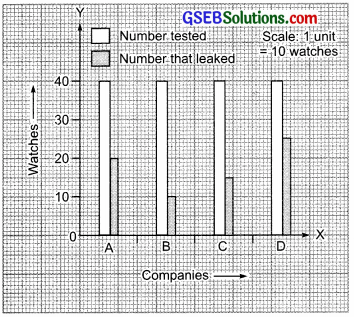Solution:
(a) From the graph, we have
∵ Total number of watches tested for each company = 40
∴ Fraction of ‘the numbers of watches that leaked’ to ‘the number of watches tested’
For company A = $$\frac { 20 }{ 40 }$$ = $$\frac { 1 }{ 2 }$$
For company B = $$\frac { 10 }{ 40 }$$ = $$\frac { 1 }{ 4 }$$
For company C = $$\frac { 15 }{ 40 }$$ = $$\frac { 3 }{ 8 }$$
For company D = $$\frac { 25 }{ 40 }$$ = $$\frac { 5 }{ 8 }$$

(b) Obviously company B has better watches.
$$\frac { 1 }{ 4 }$$ < $$\frac { 3 }{ 8 }$$ < $$\frac { 1 }{ 2 }$$ < $$\frac { 5 }{ 8 }$$

Question 2.
Sale of English and Hindi books in the year 1995, 1996, 1997 and 1998 are given below: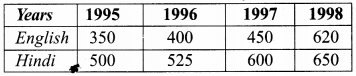Draw a double bar graph and answer the following questions:
(a) In which year was the difference in the sale of the two language books least?
(b) Can you say that the demand for English books rose faster? Justify.
Solution: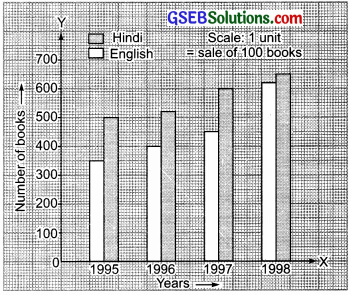(a) The difference in the sale of the two language books:
In 1995 is 500 – 350 = 150
In 1996 is 525 – 400 = 125
In 1997 is 600 – 450 = 150
In 1998 is 650 – 620 = 30
∴ The difference in the sale of the two language books is least in the year 1998.

(b) Yes, we can say that the demand of English books rose faster, because during 1995 to 1998,
(i) Sale of Hindi books rose from 500 to 650 i.e. It rose by 650 – 500 = 150
(ii) Sale of English books rose from 350 to 620 i.e. It rose by 620 – 350 = 270 270 is much greater than 150.

Question 1.
Think of some situations, atleast 3 examples of each, that are certain to happen, some that are impossible and some that may or may not happen i.e., situations that have some chance of happening.
Solution:
(i) Situations that are certain to happen:

• The sun rises in the east.
• Getting a number from 1 to 6 by throwing a dice.
• When a coin is tossed, then either a head or tail comes.

(ii) Situations that are impossible:

• Getting the number 8 by throwing a dice.
• To draw a five rupee coin from a bag containing one rupee coins.
• Drawing a red ball from a bag containing yellow and blue balls only.

(iii) Situations that have some chance of happening:

• An ant rising to 5 m height.
• To throw a dice and get an odd number.
• To toss a coin and get tail.

Note:

• A coin has two faces. The face with Ashoka Chakra is called ‘Head’ and other is called ‘Tail’.
• A cube with six faces is called a dice. Its each face has marking of dots numbering from 1 to 6.Try These (Page 75)

Question 1.
Toss a coin 100 times and record the data. Find the number of times heads and tails occur in it.
Solution:
This is a group activity. Please do it yourself.

Question 2.
Aftaab threw a die 250 times and got the following table. Draw a bar graph for this data.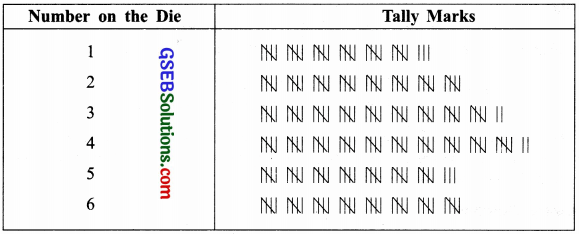Solution: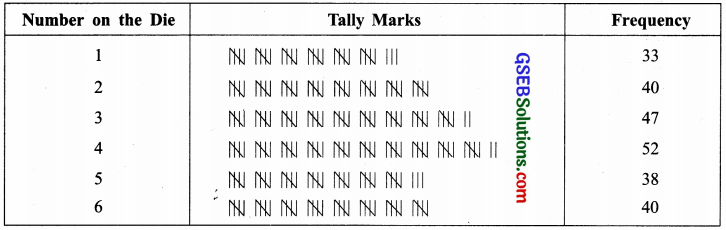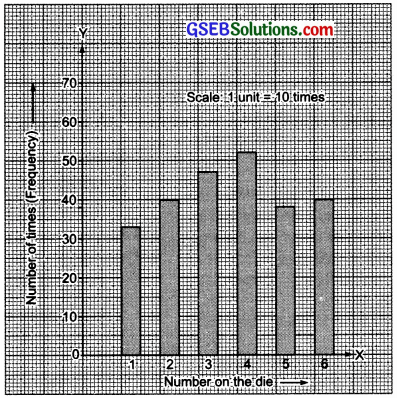Question 3.
Throw a die 100 times and record the data. Find the number of times 1, 2, 3, 4, 5, 6 occur.
Solution:
This is a group activity. Do it yourself.

Note:

• The result of effect of an action is called outcome.
• An action which results in one or several outcomes is called a trial.
• An experiment in which the result of a trial cannot be predicted in advance is called a random experiment.Try These (Page 76)

Question 1.
Construct or think of five situations where outcomes do not have equal chances.
Solution:
In the following situations, the outcomes do not have equal chances:

• To draw a red ball and to draw a yellow ball from a bag having 3 red balls and 5 yellow balls.
• To throw a dice and getting 2 and getting an odd number.
• To draw a flash card bearing ‘B’ and to draw a flash card bearing ‘C’ from a collection of 5 flash cards bearing A, B, C, D and E.
• Choosing a boy and choosing a girl as class representative from a group of 10 boys and 15 girls.
• Choosing an even number and choosing an odd number from first five natural numbers.